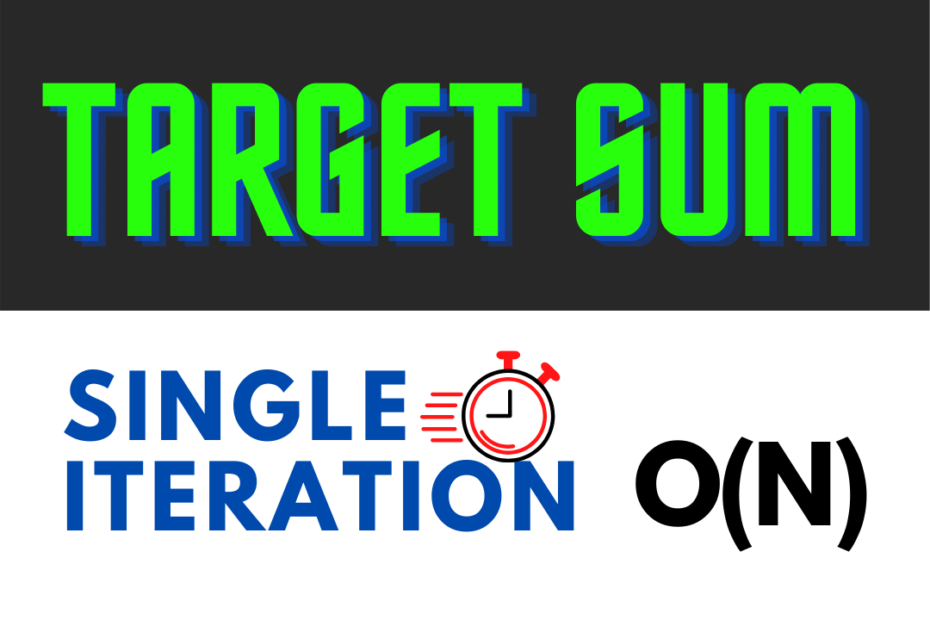# Target Sum – Single Iteration O(N)

• DSA

Given, a sorted array of elements and we need to find all the pairs of elements that add up equally to a given target sum number, this can be done usually done using two loops, like a brute force method where all the elements are compared with every other element in the array and return them if their sum is equal to the target sum.

## Traditional – Brute Force method

Uses two loops and adds every possible combination

Time Complexity: O(N2)

``````// array of numbers a
for(i=0;i<n;i++)
for(j=0;j<n;j++)
if(a[i]+a[j]==target)
print(a[i], a[j]);``````

## Optimized – Single Iteration

Why to optimize?

First of all, it’s a sorted array so the numbers are in ascending order and using this we can easily achieve the target sum.

Second, two iterations it two much. Especially in this case where we get only a few results, we are almost trying every combination and then adding them to compare.

## Explanation

For example, target = 10

``1,3,4,5,6,8,9,10,21,14``

Now, take a[i] and a[j]. i starts from the beginning and j from the end.

Now we’ll run a loop until `i<j` i.e, till we meet in the middle.

In the loop, we have three main conditions.

First if `a[i] == a[j]` print them out.

Else if `a[i]+a[j]<k` . Here the sum is less than the target, so we cannot move at the back because it’s descending order hence we increment i.

Else, it means that `a[i]+a[j]>k`. Here we crossed the target sum, which means that our element at the end is too big so now, we decrement j.

I hope you got the idea! else just have a look at the code below and perform a dry run with a pen and paper.

## Code – Target Sum

``````#include<iostream>
using namespace std;
int main()
{
int a={1,3,4,5,6,8,9,10,21,14};
// i = start, j = end and k = target
int i=0,j=10-1;
int k=10;
while(i<j)
{
if(a[i]+a[j]==k)
{
cout<<a[i]<<" + "<<a[j]<<" = "<<k<<endl;
i++;
j--;
}
else if(a[i]+a[j]<k)
i++;
else
j--;
}
return 0;
}
``````

## Output

``````1 + 9 = 10
4 + 6 = 10``````

## Conclusion

It’s a well-known fact that things become much easier when we have a sorted array…

The next time you see a sorted array, Think Twice and Iterate Once
~ Abhiram Reddy

Okay, finally I came up with a nice quote! This post is part of my #30DaysChallenge to write a blog post every day on what I learn.